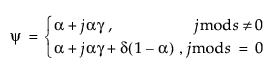Publication date: 11/29/2021

## Statistical Details for Exponential Smoothing Models

Smoothing models are defined as follows: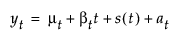where

μt is the time-varying mean term

βt is the time-varying slope term

s(t) is one of the s time-varying seasonal terms

at are the random shocks

Models without a trend have βt = 0 and nonseasonal models have s(t) = 0. The estimators for these time-varying terms are defined as follows:

Lt is a smoothed level that estimates μt

Tt is a smoothed trend that estimates βt

St - j for j = 0, 1,..., s - 1 are the estimates of the s(t)

Each smoothing model defines a set of recursive smoothing equations that describe the evolution of these estimators. The smoothing equations are written in terms of model parameters called smoothing weights:

α is the level smoothing weight

γ is the trend smoothing weight

ϕ is the trend damping weight

δ is the seasonal smoothing weight

While these parameters enter each model in a different way (or not at all), they have the common property that larger weights give more influence to recent data while smaller weights give less influence to recent data. For more information on smoothing weights, see SAS/ETS 15.2 User’s Guide (the Forecasting Process Details chapter).

### Simple Exponential Smoothing

The model for simple exponential smoothing is yt = μt + αt.

The smoothing equation, Lt = αyt + (1 – α)Lt-1, is defined in terms of a single smoothing weight α. This model is equivalent to an ARIMA(0, 1, 1) model where the following is true: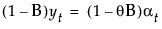where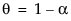The moving average form of the model is defined as follows: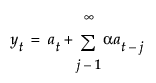### Double (Brown) Exponential Smoothing

The model for double exponential smoothing is yt = μt + β1t + at.

The smoothing equations, defined in terms of a single smoothing weight α, are defined as follows: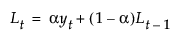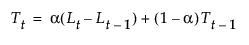This model is equivalent to an ARIMA(0, 1, 1)(0, 1, 1)1 model where the following is true: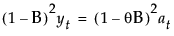where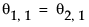withThe moving average form of the model is defined as follows: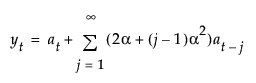### Linear (Holt) Exponential Smoothing

The model for linear exponential smoothing is yt = μt + βtt + at.

The smoothing equations, in terms of smoothing weights α and γ, are defined as follows: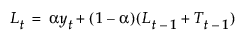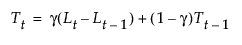This model is equivalent to an ARIMA(0, 2, 2) model where the following is true: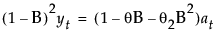with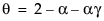and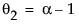The moving average form of the model is defined as follows: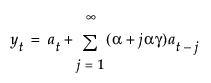### Damped-Trend Linear Exponential Smoothing

The model for damped-trend linear exponential smoothing is yt = μt + βtt + at.

The smoothing equations, in terms of smoothing weights α, γ, and ϕ, are defined as follows: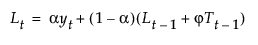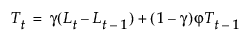This model is equivalent to an ARIMA(1, 1, 2) model where the following is true: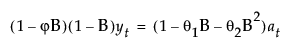where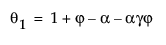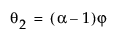The moving average form of the model is defined as follows: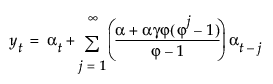### Seasonal Exponential Smoothing

The model for seasonal exponential smoothing is yt = μt + s(t) + at.

The smoothing equations in terms of smoothing weights α and δ are defined as follows: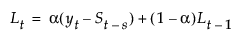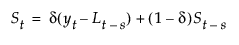This model is equivalent to a seasonal ARIMA(0, 1, s+1)(0, 1, 0)s model: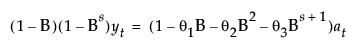where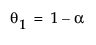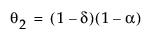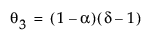The moving average form of the model is defined as follows: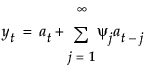where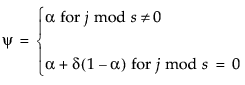The model for the additive version of the Winters method is yt = μt + βtt + s(t) + at.

The smoothing equations in terms of weights α, γ, and δ are defined as follows: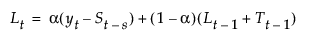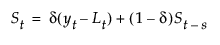This model is equivalent to a seasonal ARIMA(0, 1, s+1)(0, 1, 0)s model defined as follows: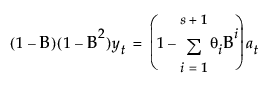The moving average form of the model is defined as follows: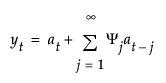where